# Handling Noise and Missing Values in Sensory Data

Sensory data are very common nowadays. Every technological device you possess it is full of sensors that you or some app can use in order to get insights about your behavior. Normally, this kind of data is called time series data because it is recorded every tot seconds. The app that you are using or you if you know how to extract this type of data, might incur in some problems give how the data is recorded.smartphone data 

It is very likely that your data contains faulty or noisy measurements that pollute your data an and hinder you from working on possible machine learning tasks you want to apply to it. For instance, GPS sensors might be imprecise and the estimated position might jump between the African continent to the American one. The same happens for accelerometers and nearly all types of sensors. In addition, some measurements could be missing, e.g. the heart rate monitor might temporarily fail. Although a variety of machine learning techniques exist that are reasonably robust against such noise, the importance of handling these issues is recognized in various research papers.

Three types of approaches exist that we can use (only on numerical attributes):

# Detecting Outliers

When you start to work with one dataset, there might be some extreme values that are very difficult to occur normally. These values are called outliers. When working with data from physical sensors, outliers are very common. Some approaches to mitigate these values can only handle single attributes, meanwhile, others can cope with complete instances.

We can have two types of outliers: those caused by a measurement error and those simply caused by the variability of the phenomenon that we observe or measure. For example, when measuring the heartbeat, a measurement of 400 would be considered a measurement error (unless the user is some kind of superhero), although a heart rate of 205 might be very uncommon but could simply be a measurement of the user trying to push his limits on a very hard sport (e.g. CrossFit). While we would clearly like to remove the measurement errors or replace them with more realistic values, we should be very careful not to remove the outliers caused by the variability in the measured quantity itself.

The easiest approach is to remove measurement errors based on domain knowledge. For example, we know that a heart rate can never be higher than 220 beats per minute and cannot be below 27 beats per minute (the current world record) . So, we remove all values outside of this range and interpret them as missing values. This will often be the right choice, but there are situations in which outliers are carrying crucial pieces of information, therefore there is the possibility that we filter out something that might change the world. Domain knowledge is not always accessible or sometimes it is unknown how to define outlier for a certain domain. When there is no domain knowledge, the outlier problem becomes an unsupervised task.

## Distribution-Based Models

One approach for outlier removal is based on the probability distribution of the data. The data should follow a standard known distribution and we remove those that are outside of certain bounds of the distribution. These approaches are mainly targeted at single attributes.

This method is called the Chauvenets criterion, where we assume that the data is following the normal distribution. The mean μ and standard deviation σ of our data are computed in order to find the normal distribution N(μ, σ²). According to Chauvenet’s criterion, a measurement from a dataset of size N is rejected when its probability of being observed is less than 1/(2N).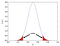We assume measurements in the red area to be outliers 

The previous approach is assuming that a single distribution can be fitted to our measurements. This is not always realistic. For example, in case of a user being active for a while and then inactive for a long time, the value from the accelerometer might follow two normal distributions, one for the active part and one for the inactive. Mixture models are created to tackle exactly this problem. The method works finding the values for the K normal distribution that best describe the data. Once the best parameters have been found, points with the lowest probabilities of being part of one of the distributions are candidates for removal.

## Distance-Based Models

Another way to detect outliers is to consider the distance between a point and the other points in the dataset. This requires a metric in order to define the distance between two instances. Various metrics have been developed for this task, but they are going to be explained in a future article. For now, just assume that we have a metric to compute the distance.

The first approach is called the Simple Distance-Based Approach, which takes a global view towards the data: it considers the distance of a point from all the other points. A certain minimum distance Dmin is given as a parameter within which we consider a point to be close to another point. If a point is distant from more than Fmin points in the dataset more than the Dmin distance, it is considered an outlier. The parameter settings for Fmin and Dmin are crucial for this approach in order to work well.

Instead of taking a global look at points the local outlier factor approach  only takes points into account that surround it. Some areas in our data space might be quite dense while others are not. Taking this into account might improve the detection of outliers. In addition, the approach specifies the likelihood of an instance to be an outlier, which is very interesting.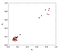Example outliers for local outlier factor 

Imagine the scenario shown in the previous figure. We can see two different clusters. One formed by data points in the top right and one formed by points in the bottom left. They are different on how dense the points are. If you consider the two black points, and you consider the shape of the two clusters, the one on the bottom left can be considered as an outlier, meanwhile, the one on the top right might be a real point in the dataset. Even though the distance from the closest point is the same, we treat them in a different way since also the structure of the closest point is important. More informations about this criterion are available in .

# Imputation of Missing Values

Obviously, our dataset could contain a lot of missing values. This could be caused by a lot of outliers that we removed in the previous step, or possibly by sensors not providing information at certain points in time, i.e. heart rate sensors recording every second meanwhile the accelerometer every millisecond. There are different ways to replace these missing values, and this process is called imputation.

One of the easiest approaches we can take is to impute the mean value of an attribute calculated over the instances where the value is known. The approach does have disadvantages when in the dataset is present data with a lot of extreme values, which they heavily impact the value of the mean. The median is a robust alternative for these cases as it is less sensitive to extreme values. For categorical values, is possible to use the mode. A more sophisticated approach is to predict the missing value for an attribute using statistical models such as linear regression. An example would be to take the previous and next value of the specific attribute and average the values. This is a simple form of linear interpolation and works under the assumption that the series follows a linear trend. This method does not work for the first and last element, which can be extrapolated using the previous or later points.

# A Combined Approach: The Kalman Filter

An approach that identifies outliers and also replaces them with new values is called the Kalman filter . The Kalman filter provides a model for the expected values based on historical data and estimates how noisy a new measurement is by comparing the observed values with the predicted values.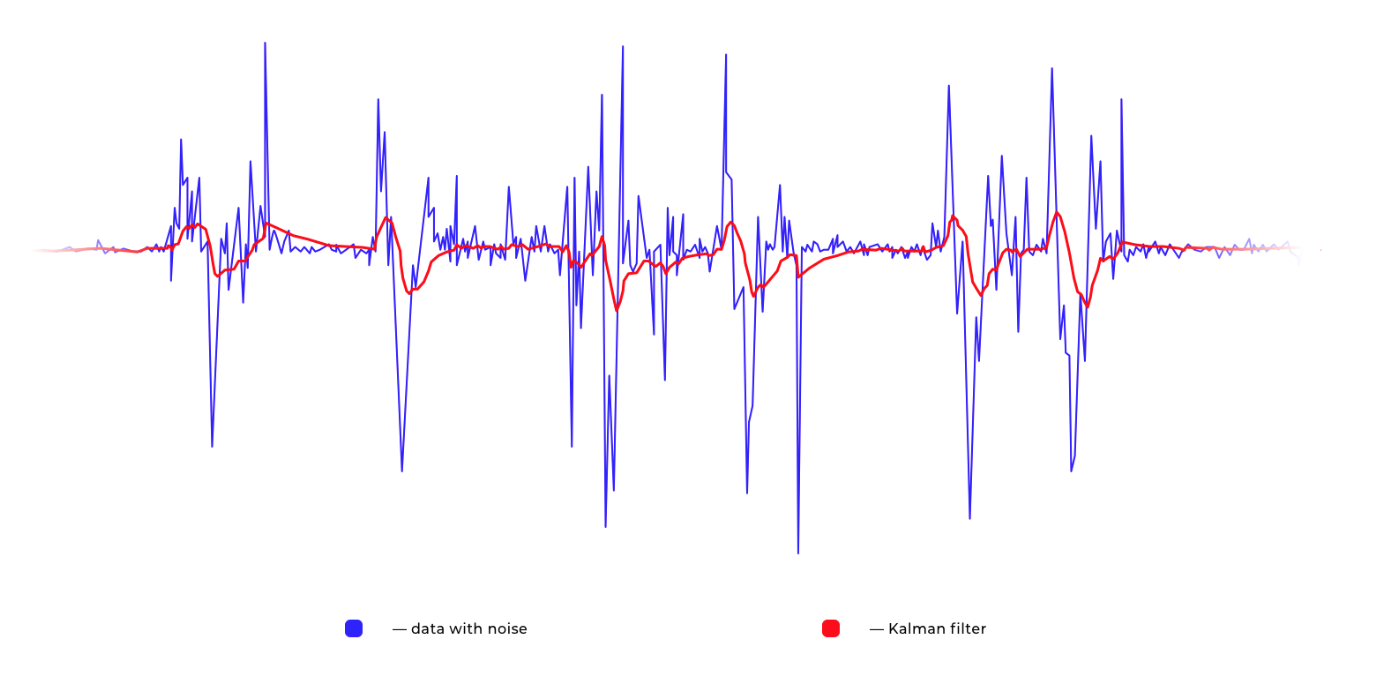Kalman Filter example 

# Transformation

The last approach for handling noisy data is to transform the data in a way that subtle noise (not the huge outliers we have seen before) is filtered and the parts of our data that explain most of the variance are identified. Two approaches are used to do this: the lowpass filter, which can be applied to individual attributes, and the Principal Component Analysis, which works across the entire dataset.

## LowPass Filter

The lowpass filter can be applied to temporal data with the assumption that there is a form of periodicity in the signal. For example, when we are walking and we are recording it with an accelerometer, periodic measurements will come out in the data at a frequency around 1Hz as walking is a repetitive pattern . This periodic parts of the data can be filtered out based on their frequency. Every measurement that comes out at a higher frequency than the walking behavior can be considered irrelevant and labeled as noise. A way to remove part of the frequency spectrum is to apply to the data the Butterworth filter. As visible in the next picture, the effect of the filter is to remove some noise from the signal, making it more meaningful for a possible machine learning algorithm.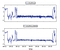Application of the filter on time series data 

## Principal Component Analysis

The Butterworth filter addresses only individual attributes, transforming the signal into the frequency domain, and then saving only a specific part of the frequency spectrum. It is also possible to consider a set of attributes at the same time and extract features that explain most of the variation observed over all attributes.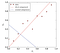Example dataset for principal component analysis 

The figure shows two attributes and the measurements from a sensor expressed by the red points. It is clearly visible that an increasing in X1 is followed by an increase of x2. In order to explain the variance of the data, the red line is computed and as visible, it describes the data in a reasonable way. Knowing the equation of the line could make us express all those points only by a single value on the line instead of a pair of values. The other line (the blue line) is a line perpendicular to the red line. It can be used as a secondary axis to express the distance of a point from our previous line. Using both, we do not lose any information, and it is possible that we get rid of the noise present in the data. This procedure is also applicable to an arbitrary number of attributes.

The main goal of Principal Component Analysis (PCA)  is to find vectors that represent these lines (or hyperplanes if we have more than two attributes) and order them in terms of how much variance in the data is explained.

# Conclusion

Now, thanks to this chapter, you should know how to handle missing values and noise in time series. If you want to read more, I definitely suggest to you , a very interesting book well written. The idea of this post comes from one of the chapters of the book. If you want to practice, the book comes with exercise and python code. Enjoy! More posts about the book are coming.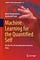The amazing book 

# References

 Hoogendoorn, M., & Funk, B. (2017). Machine Learning for the Quantified Self: On the Art of Learning from Sensory Data. Springer.

 Chauvenet, W.: A Manual of Spherical and Practical Astronomy, vol. 1, 5th ed., revised and corr. Dover Publication, New York (1960)

 Knorr, E.M., Ng, R.T.: Algorithms for mining distancebased outliers in large datasets. In: Proceedings of the International Conference on Very Large Data Bases, pp. 392–403 (1998)

 Breunig, M.M., Kriegel, H.P., Ng, R.T., Sander, J.: Lof: identifying density-based local outliers. In: ACM Sigmod Record, vol. 29, pp. 93–104. ACM (2000)

 Kalman, R.E.:Anewapproach to linear filtering and prediction problems. J. Basic Eng. 82(1), 35–45 (1960)

 Jolliffe, I.: Principal Component Analysis. Wiley Online Library, Cambridge (2002)

PhD Candidate in Artificial Intelligence @ Vrije Universiteit Amsterdam. https://www.alessandrozonta.ml/

## More from Alessandro Zonta

PhD Candidate in Artificial Intelligence @ Vrije Universiteit Amsterdam. https://www.alessandrozonta.ml/